# On the trip

On the trip were more than 55 children, but less than 65 children. Groups of 7 could be formed, but groups of 8 no. How many children were on a trip?

Correct result:

n =  63

#### Solution: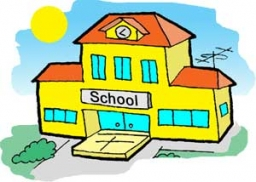We would be pleased if you find an error in the word problem, spelling mistakes, or inaccuracies and send it to us. Thank you!

Showing 1 comment:Math student
I love these math problems## Next similar math problems:

• Trio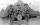56 children lined up in groups of three. How many children did not create a trio?
• GroupGroup of kids wanted to ride. When the children were divided into groups of 3 children 1 remain. When divided into groups of 4 children 1 remain. When divided into groups of 6 children 1 missed. After divided to groups of 5 children its OK. How many are t
• Dinosaurs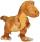More than 30 and less than 60 dinosaurs have met at the pond. A quarter of them bathed and 1/7 saws and the rest gripped. How many were at the pond? How many were there?
• Lesson exercising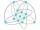The lesson of physical education, pupils are first divided into three groups so that each has the same number. The they redistributed, but into six groups. And again, it was the same number of children in each group. Finally they divided into nine equal g
• Cash back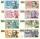After a trip 24 students have left more than 650 but less than 690 CZK (Kc). How much each student receives return when the amount is divisible by the number of pupils?
• AlcoholHow many 55% alcohol we need to pour into 14 liters 75% alcohol to get p3% alcohol? How many 65% alcohol we get?How much and how many times is 72.1 greater than 0.00721?
• Circus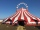On the circus performance was 150 people. Men were 10 less than women and children 50 more than adults. How many children were in the circus?
• Trams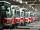Tram no. 3,7,10,11 rode together from the depot at 5am. Tram No. 3 returns after 2 hours, tram No. 7 an hour and half, no. 10 in 45 minutes and no. 11 in 30 minutes. For how many minutes and when these trams meet again?
• Roses and tulipsAt the florist are 50 tulips and 5 times less roses. How many flowers are in flower shop?
• Math classificationIn 3A class are 27 students. One-third got a B in math and the rest got A. How many students received a B in math?
• School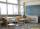27 students of 6.D class is going to a trip. They pay 9 € each. The teacher got 153 €. How many children have not paid?
• Average priceSixth grade went on a trip to Moravia. Each of the 26 pupils paid CZK 320 and the school paid a total of CZK 3,510. What was the average price of a trip per student?
• Integers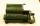May be the sum of two integers less than their difference?
• School trip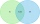On a school trip, 17 of the 28 children bought ice cream or chocolate in a candy store. Twelve children bought chocolate, and nine children bought ice cream. How many children bought ice cream and chocolate? How many children did not buy ice cream? How ma
• Multiples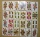What is the sum of the multiples of number 7 that are greater than 30 but less than 56?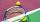The children of the tennis school received 64 white and 48 yellow balls from the sponsor. When asked about how many balls they could take, they were answered: "You have so many that none of you will have more than 10 balls and all will have the same numbe